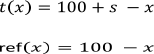QXRay Consulting

Introducing ScanDoseMatch software

#### Gamma calculationsFor every measurement point t(xi) the program calculates a series of gamma values G(xi,xm) from a partition of fitted reference values ref_fit(xm) using the expression below.where Dose Tol % is the dose tolerance in % and DTA is the distance to agreement in mm.

The calculation is performed in an interval equals twice the DTA around the measurement point xi. The resolution of the partition is one tenth of the DTA. It means for instance that when user selects a DTA of 2mm, there will be a partition of 40 points evenly distributed at 0.2mm in the ± 4mm neighborhood around the measurement point.

The reference data are interpolated with spline function to produce a series of ref_fit(xm).

The minimum of the series is assigned as the gamma index for the measurement point and displayed in the chart.

#### Validation of gamma calculations

1D Gamma index can be analytically predicted if the zero of the first derivative of the gamma function exists and it can be analytically calculated. This situation was used to validate ScanDoseMatch gamma calculations using linear datasets which describe situations similar to profile or pdd gamma calculation problems. Two sets of data and one excel file are included with the program and installed in the folder named “Test”. One dataset was created with two parallel horizontal lines separated by 1% and used to test gamma calculation in the uniform profile region. The other dataset are two parallel inclined lines with 1% shift in the y axis and were used to test gamma calculation for PDD or a simplified penumbra region.

The general problem of finding a 1D gamma index for a set of discrete values compared against a continuous function can be formulated as follows. Let t(xi) be a series of discrete values of the function t at points xi. Let ref(x) be a continuous function used as a reference function for the gamma calculation. Then consistent with the definition provided by Low et al, the gamma index Gi will be the minimum of the expression within parenthesis shown below.If the first derivative of the continuous function G(x) exists and there is a xmin that makes this derivative zero, then G(xmin) will be the gamma index at the point xi . We will accept the deviations between function t and ref at the point xi is G(xmin) is less than 1.

In the case of the two horizontal parallel lines, the tested value closer to the reference occurs exactly at x = xi. This makes the DTA section of the gamma equation zero and gamma index reduces to a relative percentage of the dose difference divided by the Dose tolerance. In our particular dataset example the gamma index becomes a constant value equal to 1%/Dose tolerance(%).

The case simulating a PDD or penumbra region it’s more complicated. Let’s assume that test and reference functions are linear as described below:where s is the percentage separation of the two functions (1 in the test case). If we define variable l as a real shift around xi but small enough compared to 100 - xi then:The above assumption is valid for points with xi lower than 90 and l in the order of one or two DTAs which is a practical condition. The gamma function G can be simplified then to:For xi <> 100 and s <> 0 then Gi has a first derivative and it is zero for the following lminwith a final location of the minimum gamma function at xi + lmin which is a gamma index G(xi + lmin) for the point xi.

The excel included with the installation implements the gamma index calculation based on this approach. Numerical results from ScanDoseMatch are in a very agreement with predicted gamma values for the points within the constraints set before.